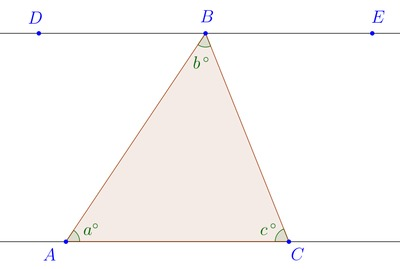# A Triangle's Interior Angles

Alignments to Content Standards: 8.G.A.5

Given that $\overleftrightarrow{DE}\parallel\overleftrightarrow{AC}$ in the diagram below, prove that $a + b + c = 180.$Explain why this result holds for any triangle, not just the one displayed above.

## IM Commentary

This problem has students argue that the interior angles of the given triangle sum to 180 degrees, and then generalize to an arbitrary triangle via an informal argument. The original argument requires students to make use the angle measure of a straight angle, and about alternate interior angles formed by a transversal cutting a pair of parallel lines (material developed experimentally via transformational geometry, e.g., in pursuing 8.G.1.b). The completion of this task, together with the explanation of how it generalizes to any triangle constitutes an informal argument (8.G.A.5) that the interior angles of any triangle add up to 180 degrees (a formal argument would involve proving from axioms and definitions that the pairs of angles used in the proof are alternate interior angles).

The task gives students to demonstrate several Practice Standards. Practice Standards SMP2 (Reason abstractly and quantitatively), SMP7 (Look for and make use of structure), and SMP8 (look for and express regularity in repeated reasoning) are all illustrated by the process of taking an initial solved problem -- in this case, the argument for the single given triangle -- and looking for the key structures that allow them to repeat that reasoning for a more abstract general setting.

## Solution

Since $\overleftrightarrow{AB}$ is a transversal of the parallel lines $\overleftrightarrow{DE}$ and $\overleftrightarrow{AC}$, we have the equality of alternate interior angles

$$\angle DBA=a$$ Similarly, using the transversal $\overleftrightarrow{BC}$ of the same lines, we have the equality of alternate interior angles $$\angle EBC=c.$$

Now since angles $\angle DBA$, $\angle EBC$, and $\angle ABC$ form a straight line, we have by substitution that

$$180 = \angle DBA + \angle ABC + \angle EBC = a + b + c,$$

and so we conclude $a+b+c=180$.

To see the result in general, take any $\triangle ABC,$ construct the parallel to $\overline{AC}$ through the point $B$. Then apply the same reasoning as above.